6.6

### 2Flonums

 (require math/flonum) package: math-lib

For convenience, math/flonum re-exports racket/flonum as well as providing the functions document below.

 procedure(fl x) → Flonum x : Real
Equivalent to (real->double-flonum x), but much easier to read and write.

Examples:
 > (fl 1/2) 0.5 > (fl 0.5) 0.5 > (fl 0.5f0) 0.5

Note that exact->inexact does not always convert a Real to a Flonum:
 > (exact->inexact 0.5f0) 0.5f0 > (flabs (exact->inexact 0.5f0)) flabs: contract violation expected: flonum? given: 0.5f0
You should prefer fl over exact->inexact, especially in Typed Racket code.

 procedure(flsgn x) → Flonum x : Flonum
 procedure(fleven? x) → Boolean x : Flonum
 procedure(flodd? x) → Boolean x : Flonum
Like sgn, even? and odd?, but restricted to flonum input.

Examples:
 > (map flsgn '(-2.0 -0.0 0.0 2.0)) '(-1.0 0.0 0.0 1.0) > (map fleven? '(2.0 1.0 0.5)) '(#t #f #f) > (map flodd? '(2.0 1.0 0.5)) '(#f #t #f)

 procedure x : Flonum
 procedure x : Flonum
 procedure(flnan? x) → Boolean x : Flonum
 procedure x : Flonum
Like rational?, infinite?, nan? and integer?, but restricted to flonum input. In Typed Racket, these are 2-3 times faster as well.

 procedure(flhypot x y) → Flonum x : Flonum y : Flonum
Computes (flsqrt (+ (* x x) (* y y))) in way that overflows only when the answer is too large.

Examples:
 > (flsqrt (+ (* 1e+200 1e+200) (* 1e+199 1e+199))) +inf.0 > (flhypot 1e+200 1e+199) 1.0049875621120889e+200

 procedure(flsum xs) → Flonum xs : (Listof Flonum)
Like (apply + xs), but incurs rounding error only once.

Examples:
 > (+ 1.0 1e-16) 1.0 > (+ (+ 1.0 1e-16) 1e-16) 1.0 > (flsum '(1.0 1e-16 1e-16)) 1.0000000000000002

The sum function does the same for heterogenous lists of reals.

Worst-case time complexity is O(n2), though the pathological inputs needed to observe quadratic time are exponentially improbable and are hard to generate purposely. Expected time complexity is O(n log(n)).

See flvector-sums for a variant that computes all the partial sums in xs.

 procedure(flsinh x) → Flonum x : Flonum
 procedure(flcosh x) → Flonum x : Flonum
 procedure(fltanh x) → Flonum x : Flonum
Return the hyperbolic sine, cosine and tangent of x, respectively.

Example:
 > (plot (list (function (compose flsinh fl) #:label "flsinh x") (function (compose flcosh fl) #:label "flcosh x" #:color 2) (function (compose fltanh fl) #:label "fltanh x" #:color 3)) #:x-min -2 #:x-max 2 #:y-label #f #:legend-anchor 'bottom-right)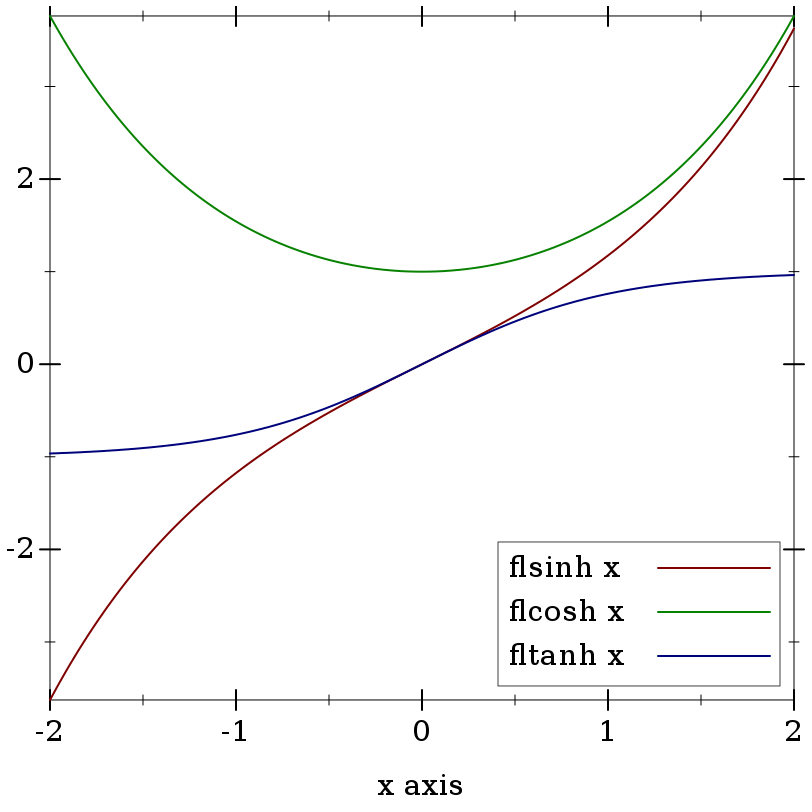Maximum observed error is 2 ulps, making these functions (currently) much more accurate than their racket/math counterparts. They also return sensible values on the largest possible domain.

 procedure(flasinh y) → Flonum y : Flonum
 procedure(flacosh y) → Flonum y : Flonum
 procedure(flatanh y) → Flonum y : Flonum
Return the inverse hyperbolic sine, cosine and tangent of y, respectively.

These functions are as robust and accurate as their corresponding inverses.

 procedure n : Flonum
 procedure(flbinomial n k) → Flonum n : Flonum k : Flonum
 procedure(flpermutations n k) → Flonum n : Flonum k : Flonum
 procedure(flmultinomial n ks) → Flonum n : Flonum ks : (Listof Flonum)
Like (fl (factorial (fl->exact-integer n))) and so on, but computed in constant time. Also, these return +nan.0 instead of raising exceptions.

For factorial-like functions that return sensible values for non-integers, see gamma and beta.

 procedure n : Flonum
 procedure(fllog-binomial n k) → Flonum n : Flonum k : Flonum
 procedure n : Flonum k : Flonum
 procedure(fllog-multinomial n ks) → Flonum n : Flonum ks : (Listof Flonum)
Like (fllog (flfactorial n)) and so on, but more accurate and without unnecessary overflow.

For log-factorial-like functions that return sensible values for non-integers, see log-gamma and log-beta.

 procedure(fllog1p x) → Flonum x : Flonum
 procedure(flexpm1 x) → Flonum x : Flonum
Like (fllog (+ 1.0 x)) and (- (flexp x) 1.0), but accurate when x is small (within 1 ulp).

For example, one difficult input for (fllog (+ 1.0 x)) and (- (flexp x) 1.0) is x = 1e-14, which fllog1p and flexpm1 compute correctly:
 > (fllog (+ 1.0 1e-14)) 9.992007221626358e-15 > (fllog1p 1e-14) 9.99999999999995e-15 > (- (flexp 1e-14) 1.0) 9.992007221626409e-15 > (flexpm1 1e-14) 1.0000000000000049e-14

These functions are mutual inverses:
 > (plot (list (function (λ (x) x) #:color 0 #:style 'long-dash) (function (compose fllog1p fl) #:label "fllog1p x") (function (compose flexpm1 fl) #:label "flexpm1 x" #:color 2)) #:x-min -4 #:x-max 4 #:y-min -4 #:y-max 4)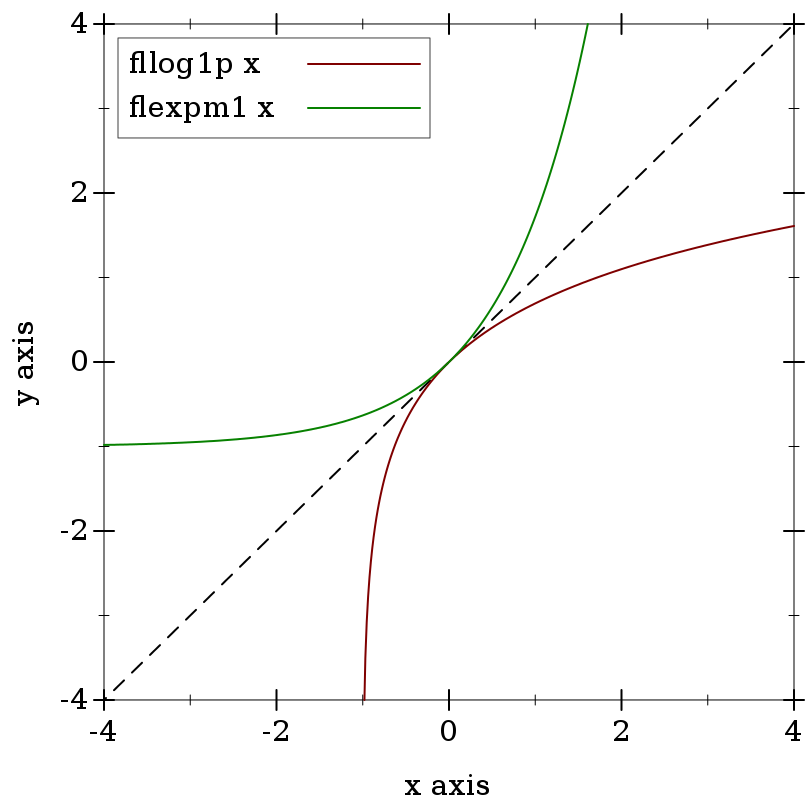Notice that both graphs pass through the origin. Thus, inputs close to 0.0, around which flonums are particularly dense, result in outputs that are also close to 0.0. Further, both functions are approximately the identity function near 0.0, so the output density is approximately the same.

Many flonum functions defined in terms of fllog and flexp become much more accurate when their defining expressions are put in terms of fllog1p and flexpm1. The functions exported by this module and by math/special-functions use them extensively.

One notorious culprit is (flexpt (- 1.0 x) y), when x is near 0.0. Computing it directly too often results in the wrong answer:
 > (flexpt (- 1.0 1e-20) 1e+20) 1.0
We should expect that multiplying a number just less than 1.0 by itself that many times would result in something less than 1.0. The problem comes from subtracting such a small number from 1.0 in the first place:
 > (- 1.0 1e-20) 1.0
Fortunately, we can compute this correctly by putting the expression in terms of fllog1p, which avoids the error-prone subtraction:
 > (flexp (* 1e+20 (fllog1p (- 1e-20)))) 0.36787944117144233
But see flexpt1p, which is more accurate still.

 procedure(flexpt1p x y) → Flonum x : Flonum y : Flonum
Like (flexpt (+ 1.0 x) y), but accurate for any x and y.

 procedure(flexpt+ x1 x2 y) → Flonum x1 : Flonum x2 : Flonum y : Flonum
Like (flexpt (+ x1 x2) y), but more accurate.

 procedure x : Flonum
Equivalent to (flexpt 2.0 x), but faster when x is an integer.

 procedure(fllog2 x) → Flonum x : Flonum
Computes the base-2 log of x more accurately than (/ (fllog x) (fllog 2.0)). In particular, (fllog2 x) is correct for any power of two x.

Examples:
 > (fllog2 4.5) 2.169925001442312 > (/ (fllog (flexp2 -1066.0)) (fllog 2.0)) -1066.0000000000002 > (fllog2 (flexp2 -1066.0)) -1066.0

Maximum observed error is 0.5006 ulps, but is almost always no more than 0.5 (i.e. it is almost always correct).

 procedure(fllogb b x) → Flonum b : Flonum x : Flonum
Computes the base-b log of x more accurately than (/ (fllog x) (fllog b)), and handles limit values correctly.

Example:
 > (plot3d (contour-intervals3d (λ (b x) (fllogb (fl b) (fl x))) 0 4 0 4) #:x-label "b" #:y-label "x")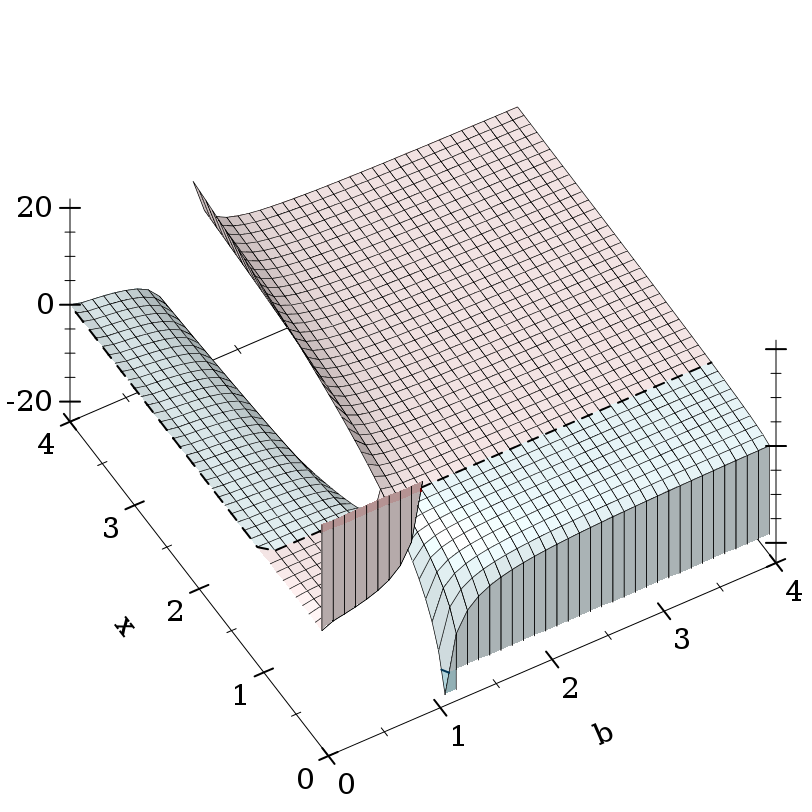Maximum observed error is 2.1 ulps, but is usually less than 0.7 (i.e. near rounding error).

Except possibly at limit values (such as 0.0 and +inf.0, and b = 1.0) and except when the inner expression underflows or overflows, fllogb approximately meets these identities for b > 0.0:

Unlike with flexpt, there is no standard for fllogb’s behavior at limit values. Fortunately, deriving the following rules (applied in order) is not prohibitively difficult.
 Case Condition Value (fllogb b 1.0) 0.0 (fllogb 1.0 x) +nan.0 (fllogb b x) b < 0.0 or x < 0.0 +nan.0 Double limits (fllogb 0.0 0.0) +inf.0 (fllogb 0.0 +inf.0) -inf.0 (fllogb +inf.0 0.0) -inf.0 (fllogb +inf.0 +inf.0) +inf.0 Limits with respect to b (fllogb 0.0 x) x < 1.0 0.0 (fllogb 0.0 x) x > 1.0 -0.0 (fllogb +inf.0 x) x > 1.0 0.0 (fllogb +inf.0 x) x < 1.0 -0.0 Limits with respect to x (fllogb b 0.0) b < 1.0 +inf.0 (fllogb b 0.0) b > 1.0 -inf.0 (fllogb b +inf.0) b > 1.0 +inf.0 (fllogb b +inf.0) b < 1.0 -inf.0
Most of these rules are derived by taking limits of the mathematical base-b log function. Except for (fllogb 1.0 x), when doing so gives rise to ambiguities, they are resolved using flexpt’s behavior, which follows the IEEE 754 and C99 standards for pow.

For example, consider (fllogb 0.0 0.0). Taking an interated limit, we get ∞ if the outer limit is with respect to x, or 0 if the outer limit is with respect to b. This would normally mean (fllogb 0.0 0.0) = +nan.0.

However, choosing +inf.0 ensures that these additional left-inverse and right-inverse identities hold:
 (fllogb 0.0 (flexpt 0.0 +inf.0)) = +inf.0 (flexpt 0.0 (fllogb 0.0 0.0)) = 0.0
Further, choosing 0.0 does not ensure that any additional identities hold.

 procedure(flbracketed-root f a b) → Flonum f : (Flonum -> Flonum) a : Flonum b : Flonum
Uses the Brent-Dekker method to find a floating-point root of f (an x : Flonum for which (f x) is very near a zero crossing) between a and b. The values (f a) and (f b) must have opposite signs, but a and b may be in any order.

Examples:
> (define (f x) (+ 1.0 (* (+ x 3.0) (sqr (- x 1.0)))))
> (define x0 (flbracketed-root f -4.0 2.0))
 > (plot (list (x-axis) (function f -4 2) (function-label f x0)) #:y-min -10)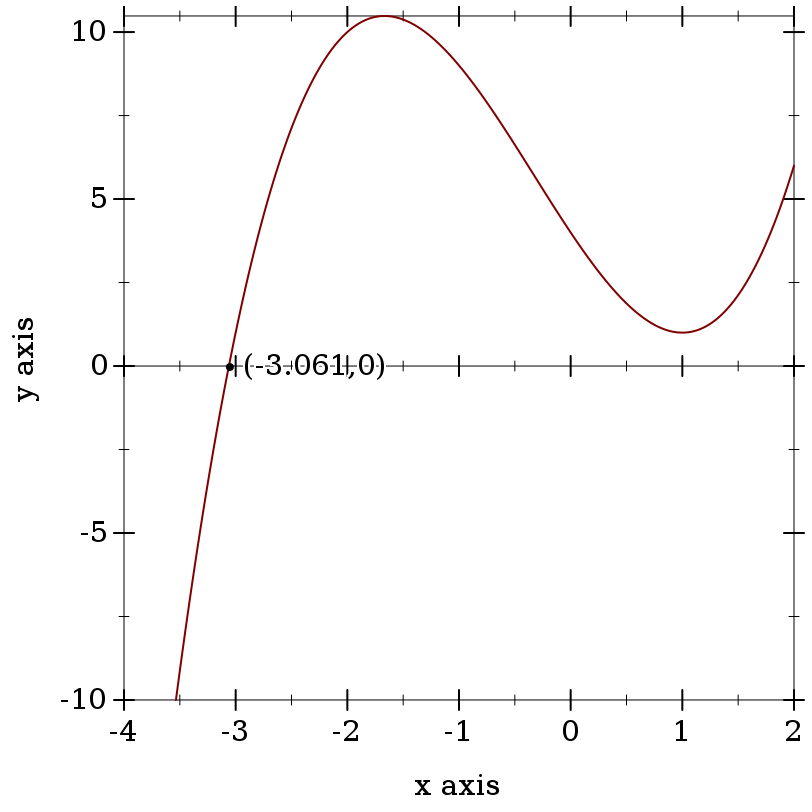> (f (flprev x0))

-7.105427357601002e-15

> (f x0)

6.661338147750939e-16

> (flbracketed-root f -1.0 2.0)

+nan.0

Caveats:
• There is no guarantee that flbracketed-root will find any particular root. Moreover, future updates to its implementation could make it find different ones.

• There is currently no guarantee that it will find the closest x to an exact root.

• It currently runs for at most 5000 iterations.

It usually requires far fewer iterations, especially if the initial bounds a and b are tight.

 procedure x : Real
Equivalent to (λ (y) (flexpt x y)) when x is a flonum, but much more accurate for large y when x cannot be exactly represented by a flonum.

Suppose we want to compute πy, where y is a flonum. If we use flexpt with an approximation of the irrational base π, the error is low near zero, but grows with distance from the origin:
 > (bf-precision 128) > (define y 150.0) > (define pi^y (bigfloat->rational (bfexpt pi.bf (bf y)))) > (flulp-error (flexpt pi y) pi^y) 43.12619934359266
Using make-flexpt, the error is near rounding error everywhere:
 > (define flexppi (make-flexpt (bigfloat->rational pi.bf))) > (flulp-error (flexppi y) pi^y) 0.8738006564073412
This example is used in the implementations of zeta and psi.

 procedure(flsqrt1pm1 x) → Flonum x : Flonum
Like (- (flsqrt (+ 1.0 x)) 1.0), but accurate when x is small.

 procedure(fllog1pmx x) → Flonum x : Flonum
Like (- (fllog1p x) x), but accurate when x is small.

 procedure(flexpsqr x) → Flonum x : Flonum
Like (flexp (* x x)), but accurate when x is large.

 procedure(flgauss x) → Flonum x : Flonum
Like (flexp (- (* x x))), but accurate when x is large.

 procedure(flexp1p x) → Flonum x : Flonum
Like (flexp (+ 1.0 x)), but accurate when x is near a power of 2.

 procedure(flsinpix x) → Flonum x : Flonum
 procedure(flcospix x) → Flonum x : Flonum
 procedure(fltanpix x) → Flonum x : Flonum
Like (flsin (* pi x)), (flcos (* pi x)) and (fltan (* pi x)), respectively, but accurate near roots and singularities. When x = (+ n 0.5) for some integer n, (fltanpix x) = +nan.0.

 procedure(flcscpix x) → Flonum x : Flonum
 procedure(flsecpix x) → Flonum x : Flonum
 procedure(flcotpix x) → Flonum x : Flonum
Like (/ 1.0 (flsinpix x)), (/ 1.0 (flcospix x)) and (/ 1.0 (fltanpix x)), respectively, but the first two return +nan.0 at singularities and flcotpix avoids a double reciprocal.

#### 2.2Log-Space Arithmetic

It is often useful, especially when working with probabilities and probability densities, to represent nonnegative numbers in log space, or as the natural logs of their true values. Generally, the reason is that the smallest positive flonum is too large.

For example, say we want the probability density of the standard normal distribution (the bell curve) at 50 standard deviations from zero:
> (require math/distributions)
> (pdf (normal-dist) 50.0)

0.0

Mathematically, the density is nonzero everywhere, but the density at 50 is less than +min.0. However, its density in log space, or its log-density, is representable:
 > (pdf (normal-dist) 50.0 #t) -1250.9189385332047
While this example may seem contrived, it is very common, when computing the density of a vector of data, for the product of the densities to be too small to represent directly.

In log space, exponentiation becomes multiplication, multiplication becomes addition, and addition becomes tricky. See lg+ and lgsum for solutions.

 procedure(lg* logx logy) → Flonum logx : Flonum logy : Flonum
 procedure(lg/ logx logy) → Flonum logx : Flonum logy : Flonum
 procedure(lgprod logxs) → Flonum logxs : (Listof Flonum)
Equivalent to (fl+ logx logy), (fl- logx logy) and (flsum logxs), respectively.

 procedure(lg+ logx logy) → Flonum logx : Flonum logy : Flonum
 procedure(lg- logx logy) → Flonum logx : Flonum logy : Flonum
Like (fllog (+ (flexp logx) (flexp logy))) and (fllog (- (flexp logx) (flexp logy))), respectively, but more accurate and less prone to overflow and underflow.

When logy > logx, lg- returns +nan.0. Both functions correctly treat -inf.0 as log-space 0.0.

To add more than two log-space numbers with the same guarantees, use lgsum.

Examples:
 > (lg+ (fllog 0.5) (fllog 0.2)) -0.35667494393873234 > (flexp (lg+ (fllog 0.5) (fllog 0.2))) 0.7000000000000001 > (lg- (fllog 0.5) (fllog 0.2)) -1.203972804325936 > (flexp (lg- (fllog 0.5) (fllog 0.2))) 0.30000000000000004 > (lg- (fllog 0.2) (fllog 0.5)) +nan.0

Though more accurate than a naive implementation, both functions are prone to catastrophic cancellation in regions where they output a value close to 0.0 (or log-space 1.0). While these outputs have high relative error, their absolute error is very low, and when exponentiated, nearly have just rounding error. Further, catastrophic cancellation is unavoidable when logx and logy themselves have error, which is by far the common case.

These are, of course, excuses—but for floating-point research generally. There are currently no reasonably fast algorithms for computing lg+ and lg- with low relative error. For now, if you need that kind of accuracy, use math/bigfloat.

 procedure(lgsum logxs) → Flonum logxs : (Listof Flonum)
Like folding lg+ over logxs, but more accurate. Analogous to flsum.

 procedure(lg1+ logx) → Flonum logx : Flonum
 procedure(lg1- logx) → Flonum logx : Flonum
Equivalent to (lg+ (fllog 1.0) logx) and (lg- (fllog 1.0) logx), respectively, but faster.

 procedure(flprobability? x [log?]) → Boolean x : Flonum log? : Any = #f
When log? is #f, returns #t when (<= 0.0 x 1.0). When log? is #t, returns #t when (<= -inf.0 x 0.0).

Examples:
 > (flprobability? -0.1) #f > (flprobability? 0.5) #t > (flprobability? +nan.0 #t) #f

#### 2.3Debugging Flonum Functions

The following functions and constants are useful in authoring and debugging flonum functions that must be accurate on the largest possible domain.

Suppose we approximate flexp using its Taylor series centered at 1.0, truncated after three terms (a second-order polynomial):
 (define (exp-taylor-1 x) (let ([x  (- x 1.0)]) (* (flexp 1.0) (+ 1.0 x (* 0.5 x x)))))

We can use plot and flstep (documented below) to compare its output to that of flexp on very small intervals:
 > (plot (list (function exp-taylor-1 #:label "exp-taylor-1 x") (function exp #:color 2 #:label "exp x")) #:x-min (flstep 1.00002 -40) #:x-max (flstep 1.00002 40) #:width 480)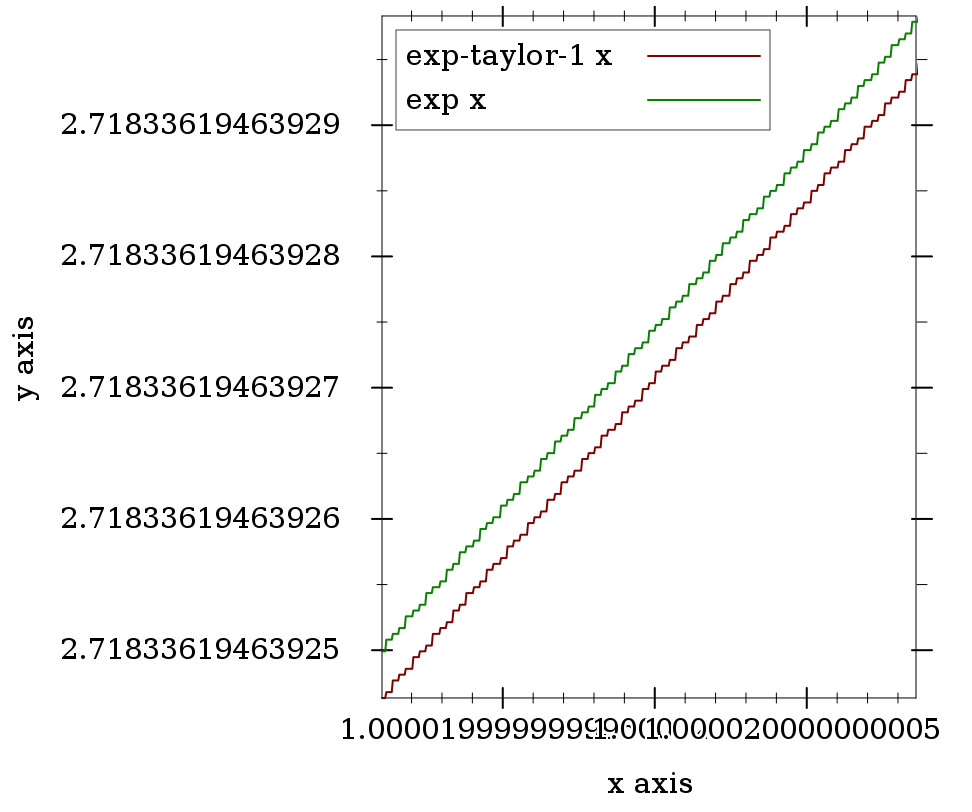Such plots are especially useful when centered at a boundary between two different approximation methods.

For larger intervals, assuming the approximated function is fairly smooth, we can get a better idea how close the approximation is using flulp-error:
 > (plot (function (λ (x) (flulp-error (exp-taylor-1 x) (exp x)))) #:x-min 0.99998 #:x-max 1.00002 #:y-label "Error (ulps)")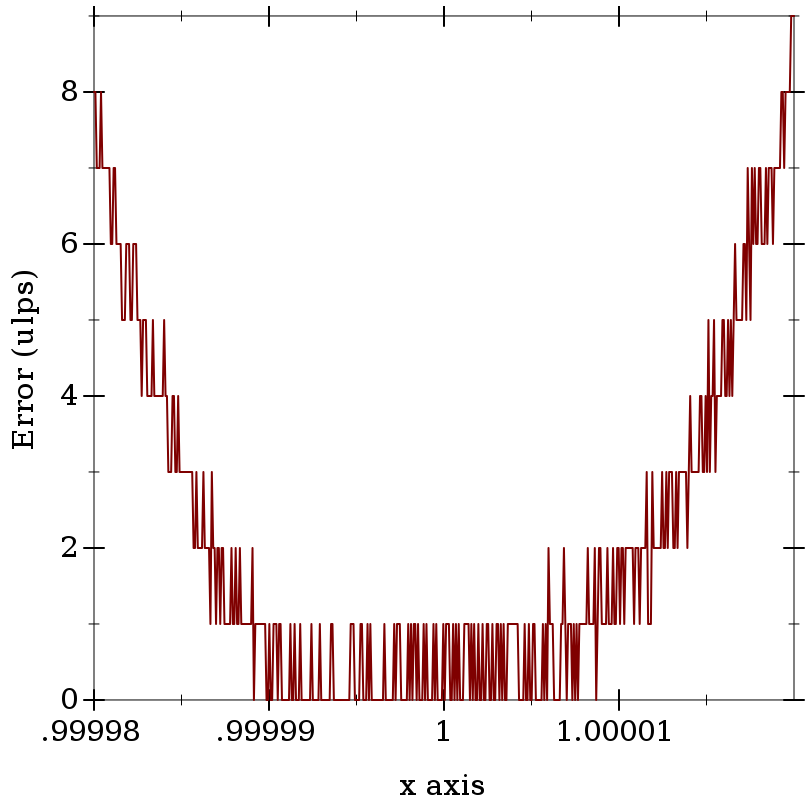We can infer from this plot that our Taylor series approximation has close to rounding error (no more than an ulp) near 1.0, but quickly becomes worse farther away.

To get a ground-truth function such as exp to test against, compute the outputs as accurately as possible using exact rationals or high-precision bigfloats.

##### 2.3.1Measuring Floating-Point Error

 procedure(flulp x) → Flonum x : Flonum
Returns x’s ulp, or unit in last place: the magnitude of the least significant bit in x.

Examples:
 > (flulp 1.0) 2.220446049250313e-16 > (flulp 1e-100) 1.2689709186578246e-116 > (flulp 1e+200) 1.6996415770136547e+184

 procedure(flulp-error x r) → Flonum x : Flonum r : Real
Returns the absolute number of ulps difference between x and r.

For non-rational arguments such as +nan.0, flulp-error returns 0.0 if (eqv? x r); otherwise it returns +inf.0.

A flonum function with maximum error 0.5 ulps exhibits only rounding error; it is correct. A flonum function with maximum error no greater than a few ulps is accurate. Most moderately complicated flonum functions, when implemented directly, seem to have over a hundred thousand ulps maximum error.

Examples:
 > (flulp-error 0.5 1/2) 0.0 > (flulp-error 0.14285714285714285 1/7) 0.2857142857142857 > (flulp-error +inf.0 +inf.0) 0.0 > (flulp-error +inf.0 +nan.0) +inf.0 > (flulp-error 1e-20 0.0) +inf.0 > (flulp-error (- 1.0 (fl 4999999/5000000)) 1/5000000) 217271.6580864

* You can make an exception when the result is to be exponentiated. If x has small absolute-error, then (exp x) has small relative-error and small flulp-error. The last example subtracts two nearby flonums, the second of which had already been rounded, resulting in horrendous error. This is an example of catastrophic cancellation. Avoid subtracting nearby flonums whenever possible.*

See relative-error for a similar way to measure approximation error when the approximation is not necessarily represented by a flonum.

##### 2.3.2Flonum Constants

 value
 value
 value
 value
The nonzero, rational flonums with maximum and minimum magnitude.

Example:
> (list -max.0 -min.0 +min.0 +max.0)
 '(-1.7976931348623157e+308 -4.9406564584125e-324 4.9406564584125e-324 1.7976931348623157e+308)

 value
The smallest flonum that can be added to 1.0 to yield a larger number, or the magnitude of the least significant bit in 1.0.

Examples:
 > epsilon.0 2.220446049250313e-16 > (flulp 1.0) 2.220446049250313e-16

Epsilon is often used in stopping conditions for iterative or additive approximation methods. For example, the following function uses it to stop Newton’s method to compute square roots. (Please do not assume this example is robust.)
 (define (newton-sqrt x) (let loop ([y  (* 0.5 x)]) (define dy (/ (- x (sqr y)) (* 2.0 y))) (if ((abs dy) . <= . (abs (* 0.5 epsilon.0 y))) (+ y dy) (loop (+ y dy)))))
When (<= (abs dy) (abs (* 0.5 epsilon.0 y))), adding dy to y rarely results in a different flonum. The value 0.5 can be changed to allow looser approximations. This is a good idea when the approximation does not have to be as close as possible (e.g. it is only a starting point for another approximation method), or when the computation of dy is known to be inaccurate.

Approximation error is often understood in terms of relative error in epsilons. Number of epsilons relative error roughly corresponds with error in ulps, except when the approximation is subnormal.

##### 2.3.3Low-Level Flonum Operations

 procedure(flonum->bit-field x) → Natural x : Flonum
Returns the bits comprising x as an integer. A convenient shortcut for composing integer-bytes->integer with real->floating-point-bytes.

Examples:
 > (number->string (flonum->bit-field -inf.0) 16) "fff0000000000000" > (number->string (flonum->bit-field +inf.0) 16) "7ff0000000000000" > (number->string (flonum->bit-field -0.0) 16) "8000000000000000" > (number->string (flonum->bit-field 0.0) 16) "0" > (number->string (flonum->bit-field -1.0) 16) "bff0000000000000" > (number->string (flonum->bit-field 1.0) 16) "3ff0000000000000" > (number->string (flonum->bit-field +nan.0) 16) "7ff8000000000000"

 procedure i : Integer
The inverse of flonum->bit-field.

 procedure x : Flonum
Returns the signed ordinal index of x in a total order over flonums.

When inputs are not +nan.0, this function is monotone and symmetric; i.e. if (fl<= x y) then (<= (flonum->ordinal x) (flonum->ordinal y)), and (= (flonum->ordinal (- x)) (- (flonum->ordinal x))).

Examples:
 > (flonum->ordinal -inf.0) -9218868437227405312 > (flonum->ordinal +inf.0) 9218868437227405312 > (flonum->ordinal -0.0) 0 > (flonum->ordinal 0.0) 0 > (flonum->ordinal -1.0) -4607182418800017408 > (flonum->ordinal 1.0) 4607182418800017408 > (flonum->ordinal +nan.0) 9221120237041090560

These properties mean that flonum->ordinal does not distinguish -0.0 and 0.0.

 procedure i : Integer
The inverse of flonum->ordinal.

 procedure x : Flonum y : Flonum
Returns the number of flonums between x and y, excluding one endpoint. Equivalent to (- (flonum->ordinal y) (flonum->ordinal x)).

Examples:
 > (flonums-between 0.0 1.0) 4607182418800017408 > (flonums-between 1.0 2.0) 4503599627370496 > (flonums-between 2.0 3.0) 2251799813685248 > (flonums-between 1.0 +inf.0) 4611686018427387904

 procedure(flstep x n) → Flonum x : Flonum n : Integer
Returns the flonum n flonums away from x, according to flonum->ordinal. If x is +nan.0, returns +nan.0.

Examples:
 > (flstep 0.0 1) 4.9406564584125e-324 > (flstep (flstep 0.0 1) -1) 0.0 > (flstep 0.0 -1) -4.9406564584125e-324 > (flstep +inf.0 1) +inf.0 > (flstep +inf.0 -1) 1.7976931348623157e+308 > (flstep -inf.0 -1) -inf.0 > (flstep -inf.0 1) -1.7976931348623157e+308 > (flstep +nan.0 1000) +nan.0

 procedure(flnext x) → Flonum x : Flonum
 procedure(flprev x) → Flonum x : Flonum
Equivalent to (flstep x 1) and (flstep x -1), respectively.

 procedure x : Flonum
Returns #t when x is a subnormal number.

Though flonum operations on subnormal numbers are still often implemented by software exception handling, the situation is improving. Robust flonum functions should handle subnormal inputs correctly, and reduce error in outputs as close to zero ulps as possible.

 value
 value
The maximum positive and negative subnormal flonums. A flonum x is subnormal when it is not zero and (<= (abs x) +max-subnormal.0).

Example:
 > +max-subnormal.0 2.225073858507201e-308

#### 2.4Double-Double Operations

For extra precision, floating-point computations may use two nonoverlapping flonums to represent a single number. Such pairs are often called double-double numbers. The exact sum of the pair is the number it represents. (Because they are nonoverlapping, the floating-point sum is equal to the largest.)

For speed, especially with arithmetic operations, there is no data type for double-double numbers. They are always unboxed: given as two arguments, and received as two values. In both cases, the number with higher magnitude is first.

Inputs are never checked to ensure they are sorted and nonoverlapping, but outputs are guaranteed to be sorted and nonoverlapping if inputs are.

 procedure(fl2 x) → (Values Flonum Flonum) x : Real (fl2 x y) → (Values Flonum Flonum) x : Flonum y : Flonum
Converts a real number or the sum of two flonums into a double-double.

Examples:
> (fl 1/7)

0.14285714285714285

> (relative-error (fl 1/7) 1/7)

5.551115123125783e-17

> (define-values (x2 x1) (fl2 1/7))
> (list x2 x1)

'(0.14285714285714285 7.93016446160826e-18)

 > (fl (relative-error (+ (inexact->exact x2) (inexact->exact x1)) 1/7))

3.0814879110195774e-33

Notice that the exact sum of x2 and x1 in the preceeding example has very low relative error.

If x is not rational, fl2 returns (values x 0.0).

 procedure(fl2->real x2 x1) → Real x2 : Flonum x1 : Flonum
Returns the exact sum of x2 and x1 if x2 is rational, x2 otherwise.

Examples:
> (define-values (x2 x1) (fl2 1/7))
> (fl2->real x2 x1)

46359793379775246683308002939465/324518553658426726783156020576256

 procedure(fl2? x2 x1) → Boolean x2 : Flonum x1 : Flonum
When x2 is rational, returns #t when (flabs x2) > (flabs x1) and x2 and x1 are nonoverlapping. When x2 is not rational, returns (fl= x1 0.0).

Examples:
> (define-values (x2 x1) (fl2 1/7))
> (fl2? x2 x1)

#t

> (fl2? 0.14285714285714285 0.07692307692307693)

#f

> (fl2? +inf.0 0.0001)

#f

This function is quite slow, so it is used only for testing.

 procedure(fl+/error x y) → (Values Flonum Flonum) x : Flonum y : Flonum
 procedure(fl-/error x y) → (Values Flonum Flonum) x : Flonum y : Flonum
 procedure(fl*/error x y) → (Values Flonum Flonum) x : Flonum y : Flonum
 procedure(fl//error x y) → (Values Flonum Flonum) x : Flonum y : Flonum
 procedure x : Flonum
 procedure x : Flonum
 procedure x : Flonum
 procedure x : Flonum
Compute the same values as (fl+ x y), (fl- x y), (fl* x y), (fl/ x y), (fl* x x), (flsqrt x), (flexp x) and (flexpm1 x), but return the normally rounded-off low-order bits as the second value. The result is an unboxed double-double.

Use these functions to generate double-double numbers directly from the results of floating-point operations.

Examples:
> (define x1 (fl 1/7))
> (define x2 (fl 1/13))
> (define z* (bigfloat->real (bfexp (bf* (bf x1) (bf x2)))))
> (relative-error (flexp (fl* x1 x2)) z*)

9.755408946378402e-17

 > (let*-values ([(y2 y1)  (fl*/error x1 x2)] [(z2 z1)  (fl2exp y2 y1)]) (fl (relative-error (fl2->real z2 z1) z*)))

4.890426935548821e-33

For flexp/error and flexpm1/error, the largest observed error is 3 ulps. (See fl2ulp.) For the rest, the largest observed error is 0.5 ulps.

 procedure(fl2zero? x2 x1) → Boolean x2 : Flonum x1 : Flonum
 procedure(fl2rational? x2 x1) → Boolean x2 : Flonum x1 : Flonum
 procedure(fl2positive? x2 x1) → Boolean x2 : Flonum x1 : Flonum
 procedure(fl2negative? x2 x1) → Boolean x2 : Flonum x1 : Flonum
 procedure(fl2infinite? x2 x1) → Boolean x2 : Flonum x1 : Flonum
 procedure(fl2nan? x2 x1) → Boolean x2 : Flonum x1 : Flonum

 procedure(fl2+ x2 x1 y2 [y1]) → (Values Flonum Flonum) x2 : Flonum x1 : Flonum y2 : Flonum y1 : Flonum = 0.0
 procedure(fl2- x2 x1 y2 [y1]) → (Values Flonum Flonum) x2 : Flonum x1 : Flonum y2 : Flonum y1 : Flonum = 0.0
 procedure(fl2* x2 x1 y2 [y1]) → (Values Flonum Flonum) x2 : Flonum x1 : Flonum y2 : Flonum y1 : Flonum = 0.0
 procedure(fl2/ x2 x1 y2 [y1]) → (Values Flonum Flonum) x2 : Flonum x1 : Flonum y2 : Flonum y1 : Flonum = 0.0
 procedure(fl2abs x2 [x1]) → (Values Flonum Flonum) x2 : Flonum x1 : Flonum = 0.0
 procedure(fl2sqr x2 [x1]) → (Values Flonum Flonum) x2 : Flonum x1 : Flonum = 0.0
 procedure(fl2sqrt x2 [x1]) → (Values Flonum Flonum) x2 : Flonum x1 : Flonum = 0.0
Arithmetic and square root for double-double flonums.

For arithmetic, error is less than 8 ulps. (See fl2ulp.) For fl2sqr and fl2sqrt, error is less than 1 ulp, and fl2abs is exact.

 procedure(fl2= x2 x1 y2 y1) → (Values Flonum Flonum) x2 : Flonum x1 : Flonum y2 : Flonum y1 : Flonum
 procedure(fl2> x2 x1 y2 y1) → (Values Flonum Flonum) x2 : Flonum x1 : Flonum y2 : Flonum y1 : Flonum
 procedure(fl2< x2 x1 y2 y1) → (Values Flonum Flonum) x2 : Flonum x1 : Flonum y2 : Flonum y1 : Flonum
 procedure(fl2>= x2 x1 y2 y1) → (Values Flonum Flonum) x2 : Flonum x1 : Flonum y2 : Flonum y1 : Flonum
 procedure(fl2<= x2 x1 y2 y1) → (Values Flonum Flonum) x2 : Flonum x1 : Flonum y2 : Flonum y1 : Flonum
Comparison functions for double-double flonums.

 procedure(fl2exp x2 x1) → (Values Flonum Flonum) x2 : Flonum x1 : Flonum
 procedure(fl2log x2 x1) → (Values Flonum Flonum) x2 : Flonum x1 : Flonum
 procedure(fl2expm1 x2 x1) → (Values Flonum Flonum) x2 : Flonum x1 : Flonum
 procedure(fl2log1p x2 x1) → (Values Flonum Flonum) x2 : Flonum x1 : Flonum
Like flexp, fllog, flexpm1 and fllog1p, but for double-double flonums.

For fl2exp and fl2expm1, error is less than 3 ulps. (See fl2ulp.) For fl2log and fl2log1p, error is less than 2 ulps.

##### 2.4.1Debugging Double-Double Functions

 procedure(fl2ulp x2 x1) → Flonum x2 : Flonum x1 : Flonum
 procedure(fl2ulp-error x2 x1 r) → Flonum x2 : Flonum x1 : Flonum r : Real
Like flulp and flulp-error, but for double-double flonums.

The unit in last place of a double-double is that of the higher-order of the pair, shifted 52 bits right.

Examples:
> (fl2ulp 1.0 0.0)

4.930380657631324e-32

 > (let-values ([(x2 x1)  (fl2 1/7)]) (fl2ulp-error x2 x1 1/7))

0.07142857142857142

 value
 value
 value
 value
The maximum-magnitude, unboxed double-double flonums.

 value
 value
The high-order flonum of the maximum-magnitude, subnormal double-double flonums.
 > +max-subnormal.0 2.225073858507201e-308 > +max-subnormal.hi 1.0020841800044864e-292
Try to avoid computing with double-doubles in the subnormal range in intermediate computations.

##### 2.4.2Low-Level Double-Double Operations

The following syntactic forms are fast versions of functions like fl+/error. They are fast because they make assumptions about the magnitudes of and relationships between their arguments, and do not handle non-rational double-double flonums properly.

 syntax
 syntax
Return two values: (fl+ x y) or (fl- x y), and its rounding error. Both assume (flabs x) > (flabs y). The values are unspecified when x or y is not rational.

 syntax(fast-fl+/error x y)
 syntax(fast-fl-/error x y)
Like fast-mono-fl+/error and fast-mono-fl-/error, but do not assume (flabs x) > (flabs y).

 syntax(fast-fl*/error x y)
 syntax(fast-fl//error x y)
 syntax
Like fl*/error, fl//error and flsqr/error, but faster, and may return garbage when an argument is subnormal or nearly infinite.

 syntax(flsplit x)
Returns nonoverlapping (values y2 y1), each with 26 bits precision, with (flabs y2) > (flabs y1), such that (fl+ y2 y1) = x. For (flabs x) > 1.3393857490036326e+300, returns (values +nan.0 +nan.0).

Used to implement double-double multiplication.

 procedure(build-flvector n proc) → FlVector n : Integer proc : (Index -> Flonum)
Creates a length-n flonum vector by applying proc to the indexes from 0 to (- n 1). Analogous to build-vector.

Example:
 > (build-flvector 10 fl) (flvector 0.0 1.0 2.0 3.0 4.0 5.0 6.0 7.0 8.0 9.0)

syntax

(inline-build-flvector n proc)

 n : Integer
 proc : (Index -> Flonum)
Like build-flvector, but always inlined. This increases speed at the expense of code size.

 procedure(flvector-map proc xs xss ...) → FlVector proc : (Flonum Flonum ... -> Flonum) xs : FlVector xss : FlVector
Applies proc to the corresponding elements of xs and xss. Analogous to vector-map.

The proc is meant to accept the same number of arguments as the number of its following flonum vector arguments. However, a current limitation in Typed Racket requires proc to accept any number of arguments. To map a single-arity function such as fl+ over the corresponding number of flonum vectors, for now, use inline-flvector-map.

syntax

(inline-flvector-map proc xs xss ...)

 proc : (Flonum Flonum ... -> Flonum)
 xs : FlVector
 xss : FlVector
Like flvector-map, but always inlined.

procedure

 (flvector-copy! dest dest-start src [ src-start src-end]) → Void
dest : FlVector
dest-start : Integer
src : FlVector
src-start : Integer = 0
src-end : Integer = (flvector-length src)
Like vector-copy!, but for flonum vectors.

 procedure vs : (Listof Real)
 procedure(flvector->list xs) → (Listof Flonum) xs : FlVector
 procedure vs : (Vectorof Real)
 procedure xs : FlVector
Convert between lists and flonum vectors, and between vectors and flonum vectors.

 procedure(flvector+ xs ys) → FlVector xs : FlVector ys : FlVector
 procedure(flvector* xs ys) → FlVector xs : FlVector ys : FlVector
 procedure(flvector- xs) → FlVector xs : FlVector (flvector- xs ys) → FlVector xs : FlVector ys : FlVector
 procedure(flvector/ xs) → FlVector xs : FlVector (flvector/ xs ys) → FlVector xs : FlVector ys : FlVector
 procedure(flvector-scale xs y) → FlVector xs : FlVector y : Flonum
 procedure(flvector-abs xs) → FlVector xs : FlVector
 procedure(flvector-sqr xs) → FlVector xs : FlVector
 procedure xs : FlVector
 procedure(flvector-min xs ys) → FlVector xs : FlVector ys : FlVector
 procedure(flvector-max xs ys) → FlVector xs : FlVector ys : FlVector
Arithmetic lifted to operate on flonum vectors.

 procedure(flvector-sum xs) → Flonum xs : FlVector
Like flsum, but operates on flonum vectors. In fact, flsum is defined in terms of flvector-sum.

 procedure xs : FlVector
Computes the partial sums of the elements in xs in a way that incurs rounding error only once for each partial sum.

Example:
 > (flvector-sums (flvector 1.0 1e-16 1e-16 1e-16 1e-16 1e+100 -1e+100))
 (flvector 1.0 1.0 1.0000000000000002 1.0000000000000002 1.0000000000000004 1e+100 1.0000000000000004)

Compare the same example computed by direct summation:
 > (rest (reverse (foldl (λ (x xs) (cons (+ x (first xs)) xs)) (list 0.0) '(1.0 1e-16 1e-16 1e-16 1e-16 1e+100 -1e+100))))

'(1.0 1.0 1.0 1.0 1.0 1e+100 0.0)